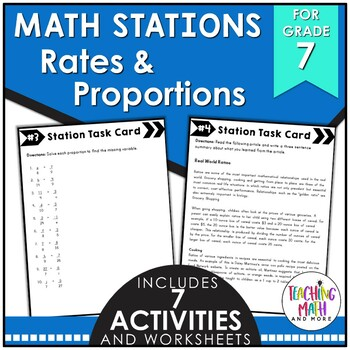## Monday, October 3, 2016

### Teaching Rates & Proportionality {Grade 7}

I LOVE teaching proportions, ratios, and rates! It's my favorite time of year when everything is equivalent and proportional. With a love for this topic I have created resources to help develop conceptual knowledge of rates and rigorous activities for practicing proportions. Here are some of my favorites!This Interactive Math Notebook activity is intended to help students understand how to compute unit rates and ratios, recognize and represent proportional relationships between quantities, decide whether two quantities are proportional, identify the unit rate, represent proportional relationships, and use proportional relationships to solve multistep ratio problems.Included are:
-4 different Rates & Proportions activities to engage students
-Teacher facilitated activity for 60-90 minutes of classroom time
-Activities include: Vocabulary, finding missing numbers and solving proportions, how to find and name equivalent ratios, and how to find and solve unit rate problems.
-Examples of Completed Interactive Math Notebook Activities are includedThis math station activity is intended to help students understand how to compute unit rates and ratios, recognize and represent proportional relationships between quantities, decide whether two quantities are proportional, identify the unit rate, represent proportional relationships, and use proportional relationships to solve multistep ratio problems.Included are:
-6 different stations to engage students
-Teacher facilitated activity for 60-90 minutes of classroom time
-Stations include:
1. Vocabulary
3. Practice of Rates & Proportionality
4. Additional Practice of Rates & Proportionality
5. Real World applications of Rates & Proportionality
6. Word problems of Rates & Proportionality
-Student Station Guide helps students record their answers{Click HERE for Unit Rates & Ratios Card Sort Activity & Assessment}
This lesson unit is intended to help you assess how well students are able to compute unit rates as they are associated with ratios of fractions, lengths, areas, and other quantities measured in different units.Included in this lesson are:
-card sort activity with concept development of ratios and unit rates
-one extension activity for students to deepen their conception of unit rates
-answer keys for all the assessments and activities
-how the lesson is tied to common core and mathematical practicesThis lesson unit is intended to help you assess how well students are able to solve problems involving scale drawings of geometric figures, including computing actual lengths and areas from a scale drawing and reproducing a scale drawing at a different scale.
Included in this lesson are:
-card sort activity with concept development of scale drawings
-one extension activity for students to deepen their conception of scale drawings
-answer keys for all the assessments and activities
-how the lesson is tied to common core and mathematical practicesThese math foldable notes are for students to be able to understand compute unit rates, ratios with fractions, measure quantities in like or different units, recognize and represent proportional relationships, decide whether two quantities are proportional, identify the constant of proportionality (unit rate), show the unit rate in tables, graphs and equations, represent proportional relationships by equations, explain points on a graph of a proportional relationship, and use proportional relationships to solve multistep ratio and percent problems.
Included are:
-7 different NO PREP Math Foldable Notes to engage students in note taking
-Teacher facilitated activity for 15-20 minutes of classroom time each
-PRINT & GO foldable notes that are ready with NO PREP
-Directions for using Math Foldable Notes in your classroom
-Options for scaffolding with blank templates
-Foldables included:
Foldable: Ratios
Foldable: Rates & Unit Rates
Foldable: Rate of Change & Slope
Foldable: Measurement Changing Units
Foldable: Solving Proportions
Foldable: Scale Drawings
Foldable: Fractions, Decimals, & Percents
Foldable: Four Blank Templates

There are lots of different approaches to teaching rates and proportions. I have found that interactive tools help the most. By using Card Sort activities you can guide and facilitate the learning process for mastery of concepts. When you find the best way to reach your students, your learning gains will increase the most!